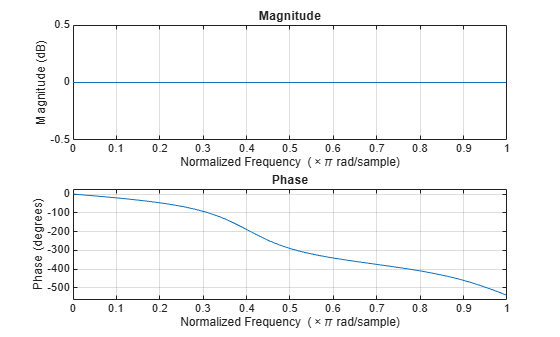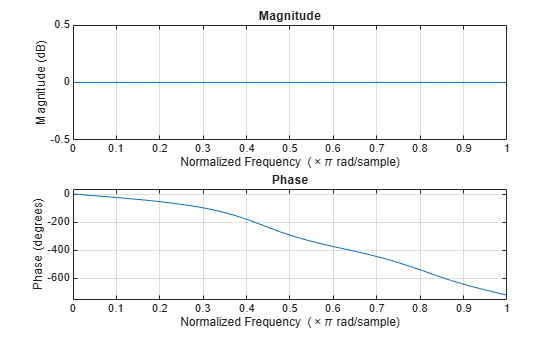# isallpass

Determine whether filter is allpass

## Syntax

```flag = isallpass(b,a) flag = isallpass(sos) flag = isallpass(d) flag = isallpass(...,tol) flag = isallpass(hs,...) flag = isallpass(hs,'Arithmetic',arithtype) ```

## Description

`flag = isallpass(b,a)` returns a logical output, `flag`, equal to `true` if the filter specified by numerator coefficients, `b`, and denominator coefficients, `a`, is an allpass filter. If the filter is not an allpass filter, `flag` is equal to `false`.

`flag = isallpass(sos)` returns `true` if the filter specified by second order sections matrix, `sos`, is an allpass filter. `sos` is a K-by-6 matrix, where the number of sections, K, must be greater than or equal to 2. Each row of `sos` corresponds to the coefficients of a second order (biquad) filter. The ith row of the `sos` matrix corresponds to ```[bi(1) bi(2) bi(3) ai(1) ai(2) ai(3)]```.

`flag = isallpass(d)` returns `true` if the digital filter, `d`, is an allpass filter. Use `designfilt` to generate `d` based on frequency-response specifications.

`flag = isallpass(...,tol)` uses the tolerance, `tol`, to determine when two numbers are close enough to be considered equal. If not specified, `tol`, defaults to `eps^(2/3)`. Specifying a tolerance may be most helpful in fixed-point allpass filters.

```flag = isallpass(hs,...)``` returns `true` if the filter System object™, `hs`, is an allpass filter. You must have the DSP System Toolbox™ software to use this syntax.

```flag = isallpass(hs,'Arithmetic',arithtype)``` analyzes the filter System object `hs` based on the specified `arithtype`. `arithtype` can be `'double'`, `'single'`, or `'fixed'`. When you specify `'double'` or `'single'`, the function performs double- or single-precision analysis. When you specify `'fixed'` , the arithmetic changes depending on the setting of the `CoefficientDataType` property and whether the System object is locked or unlocked. You must have the DSP System Toolbox software to use this syntax.

When you do not specify the arithmetic for non-CIC structures, the function uses double-precision arithmetic if the filter System object is in an unlocked state. If the System object is locked, the function performs analysis based on the locked input data type. CIC structures only support fixed-point arithmetic.

## Examples

collapse all

Create an allpass filter and verify that the frequency response is allpass.

```b = [1/3 1/4 1/5 1]; a = fliplr(b); flag = isallpass(b,a)```
```flag = logical 1 ```
`fvtool(b,a)`Create a lattice allpass filter and verify that the filter is allpass.

```k = [1/2 1/3 1/4 1/5]; [b,a] = latc2tf(k,'allpass'); flag_isallpass = isallpass(b,a)```
```flag_isallpass = logical 1 ```
`fvtool(b,a)`